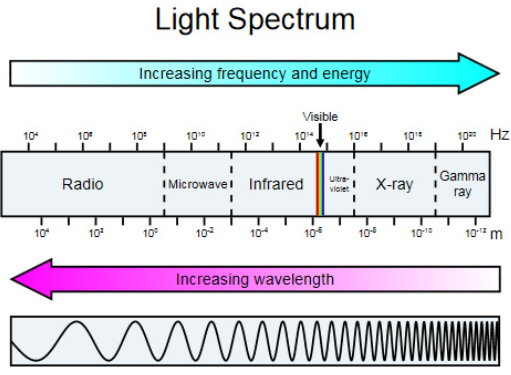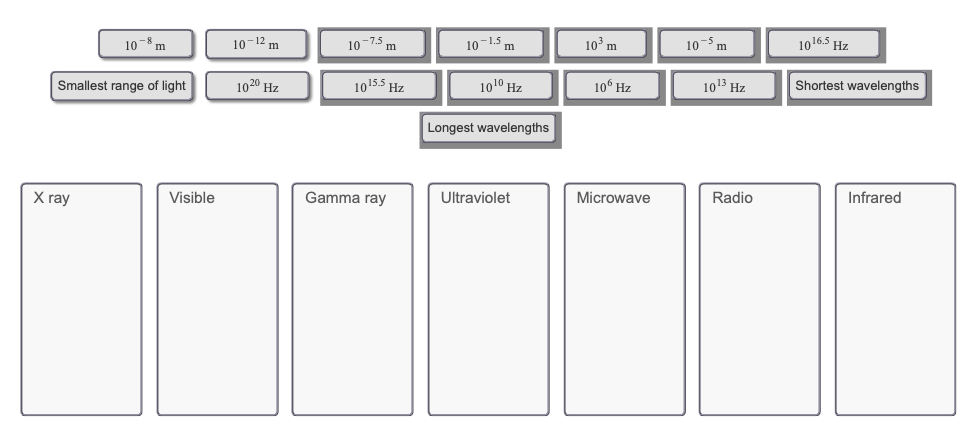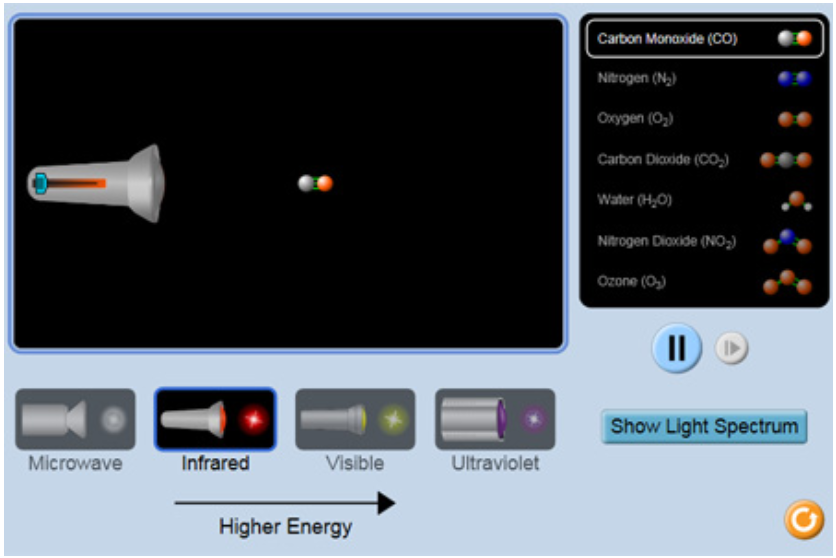# Problem: In the PhET simulation window, the following light spectrum is provided. (Access it by clicking on the gold Show Light Spectrum button in the bottom right-hand corner of the screen to open an image of the light spectrum in a new window.Look over the light spectrum and discern what information you can from its contents. Sort the following items based on what category of light they fall under or describe.Drag the appropriate items to their appropriate bins.Light, or electromagnetic radiation, exists in a variety of forms beyond what the human eye is capable of witnessing. In fact visible light makes up the smallest proportion of the light spectrum, which displays the different categories of light based on wavelength and frequency.Although there are two different ways to scientifically look at light (as a wave and as a particle), this tutorial will focus on observing light as a wave function. The wavelength of a light ray is the distance between two identical points of the wave (most commonly measured as the distance between each peak). Wavelength is commonly measured in meters on light spectra, and the wavelengths of visible light are commonly expressed in nanometers.Frequency is simply how often a particular point on the wave function is seen per unit of time. It is commonly expressed in hertz (Hz), which is a unit for cycles per second. This inverse relationship between wavelength and frequency is best explained by the speed of light equation:c = λνThe speed of light (c) is a constant equal to 2.99 × 108 m/s. Since it is a constant and a product of wavelength (λ) and frequency (ν), then increasing either wavelength or frequency must resultin a decrease of the other. This means that the longer the wavelength the lower the frequency, and the shorter the wavelength the higher the frequency.Click on the image below to explore this simulation, which shows how molecules respond to different wavelengths of light. When you click this simulation link, you may be asked whether to run, open, or save the file. Choose to run or open it.

###### FREE Expert Solution
97% (486 ratings)View Complete Written Solution
###### Problem Details

In the PhET simulation window, the following light spectrum is provided. (Access it by clicking on the gold Show Light Spectrum button in the bottom right-hand corner of the screen to open an image of the light spectrum in a new window.Look over the light spectrum and discern what information you can from its contents. Sort the following items based on what category of light they fall under or describe.

Drag the appropriate items to their appropriate bins.Light, or electromagnetic radiation, exists in a variety of forms beyond what the human eye is capable of witnessing. In fact visible light makes up the smallest proportion of the light spectrum, which displays the different categories of light based on wavelength and frequency.

Although there are two different ways to scientifically look at light (as a wave and as a particle), this tutorial will focus on observing light as a wave function. The wavelength of a light ray is the distance between two identical points of the wave (most commonly measured as the distance between each peak). Wavelength is commonly measured in meters on light spectra, and the wavelengths of visible light are commonly expressed in nanometers.

Frequency is simply how often a particular point on the wave function is seen per unit of time. It is commonly expressed in hertz (Hz), which is a unit for cycles per second. This inverse relationship between wavelength and frequency is best explained by the speed of light equation:

c = λν
The speed of light (c) is a constant equal to 2.99 × 108 m/s. Since it is a constant and a product of wavelength (λ) and frequency (ν), then increasing either wavelength or frequency must result

in a decrease of the other. This means that the longer the wavelength the lower the frequency, and the shorter the wavelength the higher the frequency.

Click on the image below to explore this simulation, which shows how molecules respond to different wavelengths of light. When you click this simulation link, you may be asked whether to run, open, or save the file. Choose to run or open it.